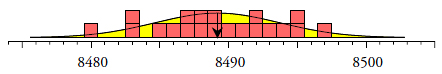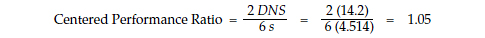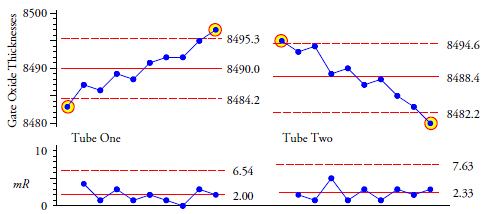#Quality Digest

Featured Product
This Week in Quality Digest Live
Standards Features
William A. Levinson
Businesses can start planning on how to meet or exceed whatever is forthcoming from OSHA
Tom Taormina
Clause 8 contains the requirements for planning, designing, and bringing to fruition products or services
Mary Rowzee
Fundamental activities of the seven-step approach
Dawn Marie Bailey
Three Baldrige Award winners share best practices
Stanley Chao
Suddenly, everybody and their brother thinks they know how to do business in China
Standards News
Good quality is adding an average of 11 percent to organizations’ revenue growth
Awards to be presented March 24, 2020, at the Quest for Excellence Conference, in National Harbor, MD
How the nation’s leading multistate cannabis company ensures quality and safety standards
New auditors must pass the exam before auditing for GFSI-recognized certification programs
ISO and WHO are working for universal access to quality health products that are all at once safe, effective, and affordable
Streamlines shop floor processes, manages nonconformance life cycle, supports enterprisewide continuous improvement
Allows construction industry to collaborate across projects and national borders
Enhances accreditation services portfolio across global market
Features illustrations as applied in real-world organizational contextsBio

Standards

## Statistics 101 and Data Analysis: an Example

### There may be a pop quiz on the secret foundation of statistical inference

Published: Monday, March 7, 2016 - 16:49

Here we look at a simple example to discover the commonalties of various data analysis techniques widely used in industry today. Careful consideration of the following may result in insights that were not part of your introductory class in statistics.

Our example uses the gate oxide thicknesses for silicon wafers. Two gate oxide tubes are used to diffuse an oxide layer onto silicon wafers. As each batch comes off each of these two production lines, a single wafer from the same location within each batch is selected, and the gate oxide thicknesses are obtained for specific locations on that wafer. The thicknesses in figure 1 are the thicknesses obtained for location number one on each of the wafers selected from 10 successive batches from each line. The target value for this gate oxide thickness is 8490 Angstroms. The specifications are 8475 to 8505 Angstroms. The values are recorded to the nearest Angstrom.Figure 1: Gate oxide thicknesses in angstroms for 10 wafers from each line

The measurement system used here has been found to possess a demonstrated resolution (probable error) of 0.9 Angstroms, thus these measurements are essentially good to the last digit.

### Statistics 101

Step 1. The first thing we are taught to do with data such as these is to compute measures of location and dispersion. For these 20 values the average is 8489.2 Angstroms, and the standard deviation statistic is 4.514 Angstroms.

Step 2. The next thing we are taught to do is to draw the histogram of the data. While there are not enough data here to even think about fitting some probability model, these statistics for location and dispersion generally give rise to a mental image something like figure 2. Based on this we might imagine that future values will be found anywhere between 8476 and 8502 Angstroms.Figure 2: Histogram and mental model for the data of figure 1

Step 3. Given the image shown in figure 2, the next thing we might think of doing is to compare the location statistic with the target value. We shall do this with a confidence interval for the mean. With a total of n = 20 data our confidence interval will have 19 degrees of freedom. The 95-percent confidence interval for the mean thickness of the gate oxides will use a Student’s t-critical value of 2.093 and will be:Since this interval contains the target value of 8490 Angstroms, we can conclude that the gate oxide tubes are operating on target (or more rigorously, we have shown that they are not detectably off-target).

Step 4. The computation above implicitly assumes that the two tubes are operating with the same average thickness. We might want to check this assumption by comparing the average thicknesses for the two tubes. We can do this by performing either a two-sample Student’s t-test, or an analysis of variance (ANOVA). Here we will do the ANOVA.

For the combined set of 20 values in figure 1, the standard deviation statistic is s = 4.51430475 Angstroms. When this value is squared and multiplied by (n–1) = 19 we have the Total Sum of Squares (TSS) for the ANOVA. Here we get a value of 387.200.

For tube one, the gate oxide thicknesses have a standard deviation statistic of s = 4.24264069. Squaring this value and multiplying by (n–1) = 9 we get 162.000. For tube two, the gate oxide thicknesses have a standard deviation statistic of s = 4.85798312. Squaring this value and multiplying by (n–1) = 9, we get 212.200. Adding these two results we get a Within Sum of Squares (WSS) of 374.400.

When we subtract the Within Sum of Squares (374.4) from the Total Sum of Squares (387.2), we get the Between Sum of Squares (BSS) of 12.800. Subtracting the Within Degrees of Freedom (18) from the Total Degrees of Freedom (19) we find that we have one degree of freedom between tubes. Finally, for the first two rows in the table below, we divide the sum of squares value by the degrees of freedom value to obtain the values in the mean squares column. Thus we get the ANOVA table shown in figure 3.Figure 3: Analysis of variance (ANOVA) for comparing tube one average with tube two average (found in figure 1)

Under the assumption that the two tubes have the same average gate oxide thicknesses, the Mean Square Between (MSB) will be an estimate of the variance parameter for the data.

At the same time, the Mean Square Within (MSW) is also an estimate of the variance parameter for the data. Since the MSW does not depend upon the tube averages, the two estimates in the mean squares column are independent of each other. So, when the two tubes have similar average values the two estimates in the mean squares column should be about the same size. We compare these two estimates by computing the ratio of the MSB to the MSW. This is known as the Fisher-ratio or F-ratio, which is 0.615 in this case.

If there is a detectable difference between the average gate oxide thicknesses for tube one and tube two, then the MSB should be noticeably larger than the MSW, and the observed F-ratio should exceed the appropriate upper-tail critical value from the F-distribution with 1 and 18 degrees of freedom. With a traditional alpha level of 5 percent, the upper-tail critical value for this case is 4.41.

Since the observed F-ratio of 0.615 is less than the critical value of 4.41 we have no detectable difference between the average gate oxide thicknesses for tube one and tube two.

Step 5. Having determined that the two tubes have similar average gate oxide thicknesses, and having determined that these two averages are reasonably close to the target thickness, we might want to assess the capability of these two gate oxide tubes. In the electronics industry this is customarily done using what the rest of the world knows as the performance ratios. For the wafers under consideration, the specifications for the gate oxide thickness are 8475 Angstroms to 8505 Angstroms. Using all 20 values from figure 1 we have a standard deviation statistic of 4.514 Angstroms, resulting in a performance ratio of:The appropriate interpretation of this ratio is that the space available within the specifications is 111 percent of the space used by this process in the past.

Incorporating the process location into the mix we have a distance to nearer specification of:So the centered performance ratio is:The appropriate interpretation of this ratio is that the effective space available within the specifications is 105 percent of the space used by this process in the past. The similarity between these two ratios once again suggests that these production lines are operating close to their target value.

Now we have computed our descriptive statistics and used them with our techniques of statistical inference to characterize this production process, which is pretty much everything we learn to do in Statistics 101. Our conclusions are:
• The two lines have the same average gate-oxide thickness.
• The two lines are operating on target.
• And the two lines are capable of operating within the specifications, but do not have much excess elbow room within the specifications.

### The foundation

Of course, everything up to this point is built upon the secret foundation of statistical inference—that ubiquitous assumption that the data are independent and identically distributed. This assumption justifies using symmetric functions of the data, and without this assumption symmetric functions of the data may well be meaningless. “What,” you may ask, “is a symmetric function of the data?” All of the computations in steps 1 through 5 above are symmetric functions of the data.

To understand this you might wish to repeat all of the computations above using the data of figure 4 where the order of the values within each tube has been shuffled. When you do these computations you will get exactly the same descriptive statistics; you will get the same histogram; you will get the same confidence interval for the mean; you will get the same ANOVA table; and you will get the same performance ratios.Figure 4: Gate oxide thicknesses in angstroms (shuffled order)

Thus, we can say that all of the computations above ignore the time-order sequence of the data. If the data are truly independent and identically distributed, then the time order is of no consequence and the values will be completely interchangeable. Thus, by using the secret foundation of statistics we greatly simplify the computational problems associated with summarizing the data.

At this point we have computed pretty much everything that can be computed. Once we assume our data are independent and identically distributed, we exhaust the possible computations fairly quickly.

About the only thing we have not yet done is to listen to the data.

### The secret of data analysis

The secret of data analysis is that your data are produced by a process rather than a probability model. Because of this, the time-order sequence of your data can contain important information that isn’t captured by symmetrical functions of the data. Returning to the data of figure 1 and using the original time-order sequences, we create separate XmR charts for the two tubes.

The average for tube one is 8490.0 and the average moving range is 2.00. Multiplying the 2.00 by the scaling factor of 2.66, and adding and subtracting the product to and from the average value we get the natural process limits of 8484.2 to 8495.3. Multiplying the 2.00 by the scaling factor of 3.27 gives the upper range limit of 6.5.

The average for tube two is 8488.4 and the average moving range is 2.33. Multiplying the 2.33 by the scaling factor of 2.66, and adding and subtracting the product to and from the average value we get the natural process limits of 8482.2 to 8494.6. Multiplying the 2.33 by the scaling factor of 3.27 gives the upper range limit of 7.6.Figure 5: XmR charts for the gate oxide thicknesses of tube one and tube two

Thus, in figure 5, in spite of the small amount of data involved, we find definite and unequivocal evidence that the gate oxide thicknesses are changing over time. Moreover, the two lines are changing in different ways. Until the assignable causes of these changes are identified, and action is taken to remove or compensate for the effects of these assignable causes from our processes, the product quality will continue to go on walkabout.

The only question that matters here is: Why are these two lines changing? By ignoring the time-order sequence in these data, the techniques of Statistics 101 failed to detect these signals of process changes, and as a result they failed to create the insight needed to ask this critical question.

Any discussion of capability, any discussion of differences between the lines, or any discussion of being on-target, is predicated upon having a predictable process. If the process is changing, then its capability, its average, and its similarity to other processes will also be changing. When the process is unpredictable, descriptive statistics and performance ratios merely describe the past without telling you anything about the future. So what do we know about these two lines?

In the past, these two production lines were near the target, but they were just driving through the neighborhood.

In the past, these two production lines were close to each other, but this was just in passing while they were driving through the neighborhood.

In the past, these two production lines had values that were within the specifications, but they just dropped in for a visit while they were in the neighborhood.

Thus we have a conundrum. All data are historical. All analyses of data are historical. But management requires prediction. So how can we use our historical data to make predictions?

The one technique that provides a basis for making predictions is a process behavior chart. When a process has been operated predictably in the past, then it’s reasonable to extrapolate from the past to the future. However, when a process has been operated unpredictably in the past, it’s not likely to spontaneously become predictable in the future. No computation exists that can compensate for the problem of having an unpredictable process. Action is required. Until the assignable causes of the unpredictable behavior have been identified and have been made part of the control strategy for that process, the past will not predict the future.

Thus, prediction requires a specific type of knowledge that the computations and techniques of Statistics 101 do not, and indeed cannot, provide. The assumption of homogeneity, which is the secret foundation of the techniques of Statistics 101, completely buries the information about the predictability, or unpredictability, of the underlying process that produces your data.Figure 6: Without homogeneity your computations become a house of cards

### Summary

By uncritically assuming your data are homogeneous you can build an elaborate house of cards with the techniques of Statistics 101. Simply dump the data into the computer and accept the resulting output as gospel. In this way, you too can learn to “lie with statistics” even without intending to do so.

Business requires prediction. Predictions require extrapolations from the past into the future. So what will be your basis for this extrapolation? You can either cross your fingers and assume the past has been homogeneous, or you can examine the data for evidence of a lack of homogeneity. Extrapolation from the past to the future only makes sense when the process producing the data has been operated predictably in the past. Everything else is wishful thinking. This is why any careful data analyst will always begin with a process behavior chart and a skeptical approach to the assumption of homogeneity for the data.

### Discuss